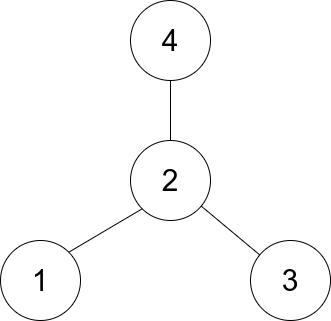# GeetCode Hub

There is an undirected star graph consisting of `n` nodes labeled from `1` to `n`. A star graph is a graph where there is one center node and exactly `n - 1` edges that connect the center node with every other node.

You are given a 2D integer array `edges` where each `edges[i] = [ui, vi]` indicates that there is an edge between the nodes `ui` and `vi`. Return the center of the given star graph.

Example 1:```Input: edges = [[1,2],[2,3],[4,2]]
Output: 2
Explanation: As shown in the figure above, node 2 is connected to every other node, so 2 is the center.
```

Example 2:

```Input: edges = [[1,2],[5,1],[1,3],[1,4]]
Output: 1
```

Constraints:

• `3 <= n <= 105`
• `edges.length == n - 1`
• `edges[i].length == 2`
• `1 <= ui, vi <= n`
• `ui != vi`
• The given `edges` represent a valid star graph.

class Solution { public int findCenter(int[][] edges) { } }